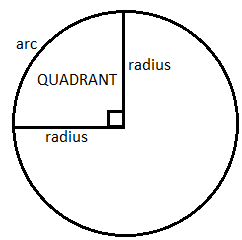QuestionAnswers

# Find the area of a quadrant of a circle whose radius is 7 centimeter.HINT:- Before solving this question, we must know about the Quadrant of a circle.
QUADRANT OF A CIRCLE: A quarter of a circle, i.e. $\dfrac{1}{4}$ of a circle, which is made by two radiuses at right angles and the connecting arc, is called a quadrant of the circle.Let us firstly calculate the area of the circle.
\begin{align} & =\pi {{r}^{2}} \\ & =\dfrac{22}{7}\times 7\times 7=154c{{m}^{2}} \\ \end{align}
Now, as we have found the area of the circle, let us divide 154 square centimeters by 4 to find the area of the quadrant of the circle.
$\dfrac{154\text{ }}{4}=\text{ }38.5\text{ }c{{m}^{2}}$
Therefore, the area of the quadrant of the circle with a radius of 7 centimeter is 38.5 square cm.
NOTE:- The students must know the formula that is used in the above solution to calculate the area of a quadrant of a circle. If the students do not know the formula to calculate the area of a quadrant of a circle, then he/she will not be able to solve this question. Here is the formula:-
To calculate the area of a quadrant of a circle, we must know the area of the circle.
We know that the area of a circle is $\pi {{r}^{2}}$ . 
Now, to calculate the area of a quadrant, we need to divide the area of the circle by 4 (as four quadrants make a circle). So, we get,
Area of a quadrant, A= $\dfrac{\left( \pi {{r}^{2}} \right)}{4}$
Now, to find the area of a quadrant of a circle, we firstly calculate the area of the circle by the formula
$\pi {{r}^{2}}$ and then we divide it by 4.

View Notes
Area of a Sector of a Circle FormulaArea of a Rhombus FormulaSurface Area of a Prism FormulaHow to Find Square Root of a NumberTo Find the Weight of a Given Body Using Parallelogram Law of VectorsThe Making of a ScientistChanging the Period of a PendulumArea of TrapeziumArea of Hollow CylinderArea of Triangle FormulaImportant Questions for CBSE Class 6 English A Pact with The Sun Chapter 1 - A Tale of Two BirdsImportant Questions for CBSE Class 6 English A Pact with The Sun Chapter 10 - A Strange Wrestling MatchImportant Questions for CBSE Class 6 English A Pact with The Sun Chapter 8 - A Pact with the SunImportant Questions for CBSE Class 6 English Honeysuckle Chapter 8 - A Game of ChanceImportant Questions for CBSE Class 11 Business Studies Chapter 7 - Formation of a CompanyImportant Questions for CBSE Class 7 English Honeycomb Chapter 2 - A Gift Of ChappalsImportant Questions for CBSE Class 6 English Honeysuckle Chapter 5 - A Different Kind of SchoolImportant Questions for CBSE Class 8 Social Science Our Past 3 Chapter 4 - Tribals, Dikus and the Vision of a Golden AgeImportant Questions for CBSE Class 7 English Honeycomb Chapter 10 - The Story of CricketImportant Questions for CBSE Class 6 English A Pact with The SunCBSE Class 10 Hindi A Question Paper 2020Hindi A Class 10 CBSE Question Paper 2009Hindi A Class 10 CBSE Question Paper 2015Hindi A Class 10 CBSE Question Paper 2016Hindi A Class 10 CBSE Question Paper 2012Hindi A Class 10 CBSE Question Paper 2010Hindi A Class 10 CBSE Question Paper 2007Hindi A Class 10 CBSE Question Paper 2013Hindi A Class 10 CBSE Question Paper 2008Hindi A Class 10 CBSE Question Paper 2014RS Aggarwal Class 8 Mathematics Solutions for Chapter-18 Area of a Trapezium and a PolygonRD Sharma Class 7 Solutions Chapter 20 - Mensuration I (Area of Circle) (Ex 20.2) Exercise 20.2RS Aggarwal Solutions Class 8 Chapter-18 Area of a Trapezium and a Polygon (Ex 18B) Exercise 18.2RD Sharma Class 7 Solutions Chapter 20 - Mensuration I (Area of Circle) (Ex 20.1) Exercise 20.1RD Sharma Solutions for Class 9 Maths Chapter 21 - Surface Area and Volume of a SphereRS Aggarwal Solutions Class 8 Chapter-18 Area of a Trapezium and a Polygon (Ex 18A) Exercise 18.1RS Aggarwal Solutions Class 8 Chapter-18 Area of a Trapezium and a Polygon (Ex 18C) Exercise 18.3RD Sharma Solutions for Class 9 Maths Chapter 19 - Surface Area and Volume of A Right Circular CylinderRD Sharma Solutions for Class 9 Maths Chapter 18 - Surface Area and Volume of a Cuboid and CubeRD Sharma Solutions for Class 9 Maths Chapter 20 - Surface Area and Volume of A Right Circular Cone